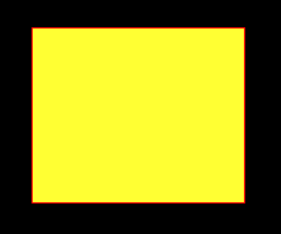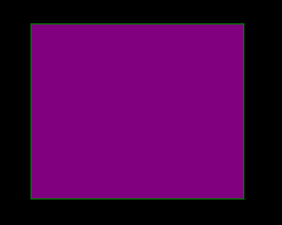GeeksforGeeks App
Open AppBrowser
Continue

# Python PIL | ImageDraw.Draw.rectangle()

PIL is the Python Imaging Library which provides the python interpreter with image editing capabilities. The `ImageDraw` module provide simple 2D graphics for Image objects. You can use this module to create new images, annotate or retouch existing images, and to generate graphics on the fly for web use.

`ImageDraw.Draw.rectangle()` Draws an rectangle.

Syntax: PIL.ImageDraw.Draw.rectangle(xy, fill=None, outline=None)
Parameters:

xy – Four points to define the bounding box. Sequence of either [(x0, y0), (x1, y1)] or [x0, y0, x1, y1]. The second point is just outside the drawn rectangle.
outline – Color to use for the outline.
fill – Color to use for the fill.

Returns: An Image object in rectangle shape.

 `  ` ` ` `# importing image object from PIL``import` `math``from` `PIL ``import` `Image, ImageDraw`` ` `w, h ``=` `220``, ``190``shape ``=` `[(``40``, ``40``), (w ``-` `10``, h ``-` `10``)]`` ` `# creating new Image object``img ``=` `Image.new(``"RGB"``, (w, h))`` ` `# create rectangle image``img1 ``=` `ImageDraw.Draw(img)  ``img1.rectangle(shape, fill ``=``"# ffff33"``, outline ``=``"red"``)``img.show()`

Output:Another Example: Here we use different colour for filling.

 `  ` ` ` `# importing image object from PIL``import` `math``from` `PIL ``import` `Image, ImageDraw`` ` `w, h ``=` `220``, ``190``shape ``=` `[(``40``, ``40``), (w ``-` `10``, h ``-` `10``)]`` ` `# creating new Image object``img ``=` `Image.new(``"RGB"``, (w, h))`` ` `# create  rectangleimage``img1 ``=` `ImageDraw.Draw(img)  ``img1.rectangle(shape, fill ``=``"# 800080"``, outline ``=``"green"``)``img.show()`

Output:My Personal Notes arrow_drop_up